## A body of mass 5 kg moving with velocity 10m/s attains a velocity of 30m/s in 20 seconds, after applying the force. The amount of force appl

Question

A body of mass 5 kg moving with velocity 10m/s attains a velocity of 30m/s in 20 seconds, after applying the force. The amount of force applied will be:​

in progress 0
6 months 2021-07-16T20:43:07+00:00 1 Answers 17 views 0

## Answers ( )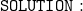• We’re provided – mass ( m ) = 5 kg , initial velocity ( u ) = 10 m / s , final velocity ( v ) = 30 m / s , Time taken ( t ) = 20 sec and we’re asked to find out the amount of force applied.
• Here , we’ll use ‘ F = ma ‘ in order to find out the amount of force applied where ‘ F ‘ refers to Force applied , ‘ m’ refers to mass of the body and ‘ a ‘ refers to acceleration.
• Since we aren’t provided the acceleration , we’re gonna use ‘ v – u / t ‘ where ‘ v ‘ refers to final velocity , ‘ u ‘ refers to initial velocity & ‘ t ‘ refers to time taken.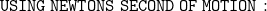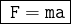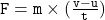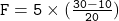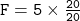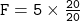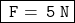• Hence , the amount of force applied will be 5 Newton .

Hope I helped! Let me know if you have any queries regarding my answer and also , Notify me if you need any other help ! 🙂

▁▁▁▁▁▁▁▁▁▁▁▁▁▁▁▁▁▁▁▁▁▁▁▁▁▁